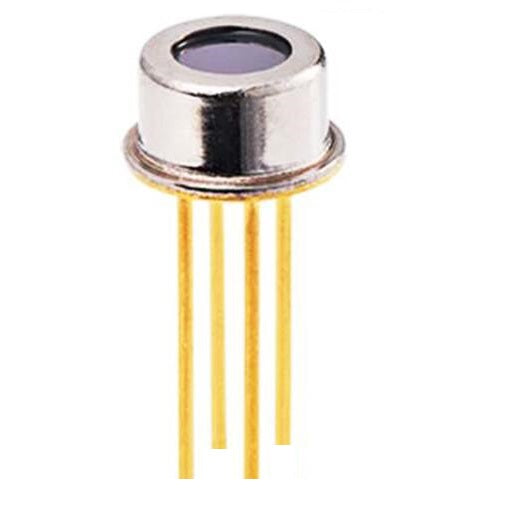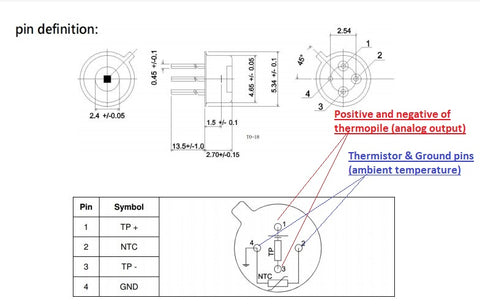1

#### Future Electronics Egypt# Analog Thermopile Contactless Infrared Temperature

## LE 115.00

Thermopiles are used to measure temperature from a distance by detecting an object's infrared (IR) energy. The higher the temperature, the more IR energy is emitted. The thermopile sensing element, composed of small thermocouples on a silicon chip, absorb the energy and produce an output signal. This ZTP-135SR thermopile infrared sensor consists of thermo-elements, flat IR filter and a thermistor for temperature compensation.

Thermopile Working Principle and Circuit

The thermopile infrared temperature sensor module has 4 pins: 2 for thermistor (ambient temperature) measurements and the other 2 pins are for the thermopile (object temperature) measurements as shown in figure.

The thermopile signal (voltage output) is a small signal and should be amplified (see tutorials below). Once the voltage output from thermopile is measured (V) and Ambient temperature from thermistor measured, then we might use the following equation to calculate the object temperature (TO).

V = S.(TO^B – TA^B)

• V = Output voltage from thermopile (measured by microcontroller)
• TO = Object temperature in K
• TA = Ambient temperature in K (measured by thermistor)
• S = Sensitivity coefficient (from datasheet)
• B = Coefficient (~4)
•Featrures

• Object temperature: -20 to +100°C
• Fast response time
• Analog output
• Thermister output for ambient temperature (referrence temperature)
• Low cost, make it favourable choise medical and industrial applications such as thermometer guns

Thermopile contactless infrared temperature sensor (ZTP-135SR) Datasheet.

Instructables on how to use thermopile infrared sensor including circuit and Arduino code.

More notes on practical use of  thermopile sensor.

Sale

Unavailable

Sold Out